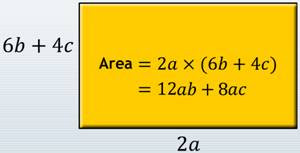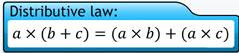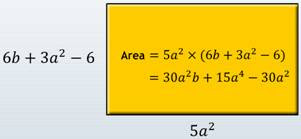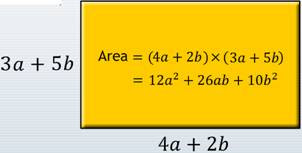## 12 September 2014

### Algebraic Identities

An equation is true for only certain values of the variables and need not be true for all values of the variables. If it is true for all values of the variables in it, then it is called an Identity.

These identities are obtained on multiplying one binomial with another binomial and are known as Standard Identities.

In the previous post , we have learned about , and their addition and subtraction, monomials, binomials, trinomials, etc.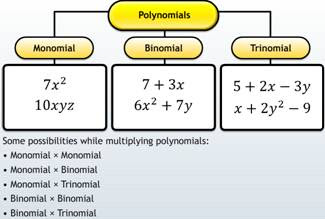The product of monomials is equal to the product of their coefficients multiplied by the product of their variables.
Product of Monomials = Product of Coefficients x Product of Variables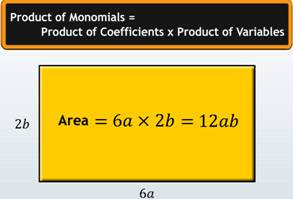To multiply polynomials, you have to:
1. Apply the distributive law to reduce the multiplication of expressions to products of monomials.
2. Add the powers of the variable, if a variable is multiplied with itself.
3. Add the like terms in the product.